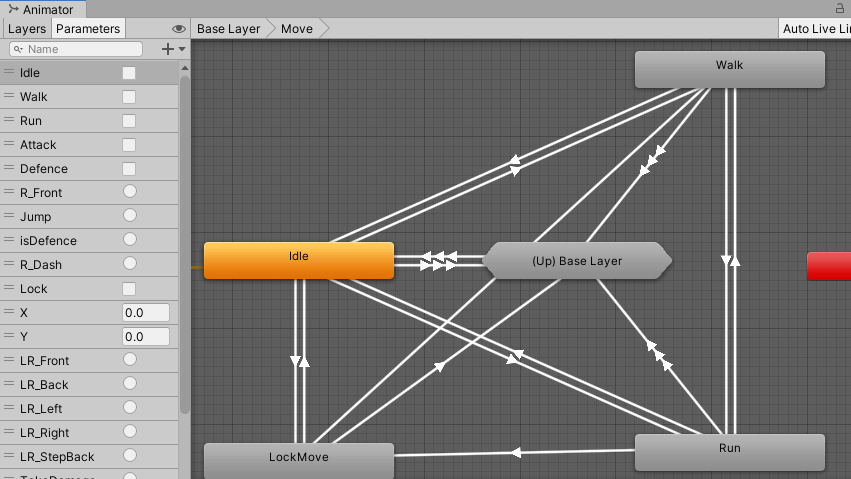## 前言

（这个是我自研的方法，肯定不会是最好的方法，仅供思路参考）

## 需要解决的问题

• 角色转向时的旋转问题
• 角色移动时需要参考摄像机的位置移动
• 摄像机所给出的角度需要垂直于地面
• 当角色180°的大旋转时的急停过渡动画如何使用

## 方法参考

### 角色转向时的旋转问题``transform.rotation = Quaternion.Lerp(transform.rotation, Quaternion.LookRotation(Camera.main.transform.forward), 15f * Time.deltaTime);```

## 代码部分

`注:按键获取使用的是Input System的方法`

``````private void move() //真旋转版
{
if (isRotate)
{
//moveValue.x = Mathf.Lerp(moveValue.x, moveInput.x, 0.05f);
//moveValue.z = Mathf.Lerp(moveValue.z, moveInput.y, 0.05f);
if (keyboard.wKey.isPressed)
{
transform.Translate(Vector3.ProjectOnPlane(Camera.main.transform.forward, Vector3.up).normalized * Time.deltaTime * moveSpeed * moveInput.y, Space.World);
transform.rotation = Quaternion.Lerp(transform.rotation, Quaternion.LookRotation(Camera.main.transform.forward), 15f * Time.deltaTime);
}
if (keyboard.sKey.isPressed)
{              transform.Translate(Vector3.ProjectOnPlane(Camera.main.transform.forward, Vector3.up).normalized * Time.deltaTime * moveSpeed * moveInput.y, Space.World);
transform.rotation = Quaternion.Lerp(transform.rotation, Quaternion.LookRotation(-Camera.main.transform.forward), 15f * Time.deltaTime);
}
if (keyboard.aKey.isPressed)
{         transform.Translate(Vector3.ProjectOnPlane(Camera.main.transform.right, Vector3.up).normalized * Time.deltaTime * moveSpeed * moveInput.x, Space.World);
transform.rotation = Quaternion.Lerp(transform.rotation, Quaternion.LookRotation(-Camera.main.transform.right), 10f * Time.deltaTime);
}
if (keyboard.dKey.isPressed)
{
transform.Translate(Vector3.ProjectOnPlane(Camera.main.transform.right, Vector3.up).normalized * Time.deltaTime * moveSpeed * moveInput.x, Space.World);
transform.rotation = Quaternion.Lerp(transform.rotation, Quaternion.LookRotation(Camera.main.transform.right), 10f * Time.deltaTime);
}
}
}
``````